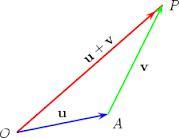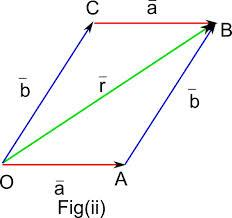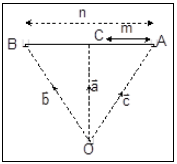Courses

# Notes | EduRev

## JEE: Notes | EduRev

The document Notes | EduRev is a part of the JEE Course JEE Revision Notes.
All you need of JEE at this link: JEE
• The length or the magnitude of the vector  = (a, b, c) is defined by w = √a2+b2+c2
• A vector may be divided by its own length to convert it into a unit vector, i.e. ? = u / |u|. (The vectors have been denoted by bold letters.)
• If the coordinates of point A are xA, yA, zA and those of point B are xB, yB, zB then the vector connecting point A to point B is given by the vector r, where r = (xB - xA)i + (yB – yA) j + (zB – zA)k , here i, j and k denote the unit vectors along x, y and z axis respectively.
• Some key points of vectors:
1) The magnitude of a vector is a scalar quantity
2) Vectors can be multiplied by a scalar. The result is another vector.
3) Suppose c is a scalar and v = (a, b) is a vector, then the scalar multiplication is defined by cv = c (a, b) = (ca, cb). Hence each component of vector is multiplied by the scalar.
4) If two vectors are of the same dimension then they can be added or subtracted from each other. The result is gain a vector.
• If u, v and w are three vectors and c, d are scalars then the following results of vector addition hold true:
1) u + v = v + u (the commutative law of addition)
2) u + 0 = u
3) u + (-u) = 0 (existence of additive inverses)
4) c (du) = (cd)u
5) (c + d)u = cu + d u
6) c(u + v) = cu + cv
7) 1u = u
8) u + (v + w) = (u + v) + w (the associative law of addition)
• Given two vectors u and v, their sum or resultant written as (u+v) is also a vector obtained by first bringing the initial point of v to the terminal point of u and then joining the initial point of u to the terminal point of v giving a consistent direction by completing the triangle OAP. This is termed as the Triangle Law of Addition.• Let a and b be any two vectors. From the initial point of a, vector b is drawn and the parallelogram AOCB is completed with OA and OB as adjacent sides. The vector OC is defined as the sum of a and b. This is called the parallelogram law of vectors.• For adding more than two vectors, Polygon Law of Addition is used.
• Internal and External Division:
1) If A and B are two points with position vectors a and b respectively and C is a point which divides AB internally in the ratio m : n, then the position vector of C is given by OC = (mb + na)/ (m + n). This is termed as internal division.
2) If A and B are two points with position vectors a and b respectively and C is a point which divides AB externally in the ratio m : n, then the position vector of C is given by OC = (mb - na)/ (m-n)3) In case, C is the mid-point of AB, then the position vector of C is (a + b)/2.
4) The position vector of any point C on AB can always be assumed as c = la + mb, where l + m = 1.
• If circumcentre is origin and vertices of a triangle have position vectors a, b and c, then the position vector of orthocentre will be -(a+b+c).
• a.b is the product of length of one vector and length of the projection of the other vector in the direction of former.
• a.b = |a| Projection of |b| in direction of a = |b|. Projection of |a| in direction of b.
• Some Basic Rules of Algebra of Vectors:
1) a.a = |a|2 = a2
2) a.b = b.a
3) a.0 = 0
4) a.b = (a cos q)b = (projection of a on b)b = (projection of b on a) a
5) a.(b + c) = a.b + a.c (This is also termed as the distributive law)
6) (la).(mb) = lm (a.b)
7) (a ± b)2 = (a ± b) . (a ± b) = a+ b2 ± 2a.b
8) If a and b are non-zero, then the angle between them is given by cos θ = a.b/|a||b|
9) a x a = 0
10) a x b = - (b x a)
11) a x (b + c) = a x b + a x c
• Any vector perpendicular to the plane of a and b is l(a x b) where l is a real number.
• Unit vector perpendicular to a and b is ± (a x b)/ |a x b|
• The position of dot and cross can be interchanged without altering the product. Hence it is also represented by [a b c]
1) [a b c] = [b c a] = [c a b]
2) [a b c] = - [b a c]
3) [ka b c] = k[a b c]
4) [a+b c d] = [a c d] + [b c d]
5) a x (b x c) = (a x b) x c, if some or all of a, b and c are zero vectors or a and c are collinear.
• Methods to prove collinearity of vectors:
1) Two vectors a and b are said to be collinear if there exists k ? R such that a = kb.
2) If p x q = 0, then p and q are collinear.
3) Three points A(a), B(b) and C(c) are collinear if there exists k ? R such that AB = kBC i.e. b-a = k (c-b).
4) If (b-a) x (c-b) = 0, then A, B and C are collinear.
5) A(a), B(b) and C(c) are collinear if there exists scalars l, m and n (not all zero) such that la + mb+ nc = 0, where l + m + n = o
• Three vectors p, q and r are coplanar if there exists l, m ? R such that r = lp + mq i.e., one can be expressed as a linear combination of the other two.
• If [p q r] = 0, then p, q and r are coplanar.
• Four points A(a), B(b), C(c) and D(d) lie in the same plane if there exist l, m ? R such that b-a = l(c-b) + m(d-c).
• If [b-a c-b d-c] = 0 then A, B, C, D are coplanar.
• Two lines in space can be parallel, intersecting or neither (called skew lines). Let r = a1 + μb and r = a2 + μb2 be two lines.
• They intersect if (b1 x b2)(a- a1) = 0
• The two lines are parallel if b1 and b2 are collinear.
• The angle between two planes is the angle between their normal unit vectors i.e. cos q = n1 . n2
• If a, b and c are three coplanar vectors, then the system of vectors a', b' and c' is said to be the reciprocal system of vectors if aa' = bb' = cc' = 1  where a' = (b xc) /[a b c] , b' = (c xa)/ [a b c] and c' = (a x b)/[a b c] Also, [a' b' c'] = 1/ [a b c]
• Dot Product of two vectors a and b defined by a = [a1, a2, ..., an] and b = [b1, b2, ..., bn] is given by a1b1  + a2b+ ...,  + anbn
• Some Basic Results of Vector Calculus:
1) Vectors in the same direction can be added by simply adding their magnitudes. But if the vectors to be added are in opposite directions, then their magnitudes are subtracted and not added.
2) Column vectors can be added by simply adding the values in each row.
3) You can find the magnitude of a vector in three dimensions by using the formula a2 = b+ c2 + d2, where a is the magnitude of the vector, and b, c, and d are the components in each direction.
4) If l1a + m1b = l2a + m2b then l1 = l2 and m= m2
5) Collinear Vectors are also parallel vectors except that they lie on the same line.
6) When two vectors are parallel, the dot product of the vectors is 1 and their cross product is zero.
7)Two collinear vectors are always linearly dependent.
8) Two non-collinear non-zero vectors are always linearly independent
9) Three coplanar vectors are always linearly dependent.
10) Three non-coplanar non-zero vectors are always linearly independent.
11) More than 3 vectors are always linearly dependent.
12) Three vectors are linearly dependent if they are coplanar that means any one of them can be represented as a linear combination of other two.
The document Notes | EduRev is a part of the JEE Course JEE Revision Notes.
All you need of JEE at this link: JEEUse Code STAYHOME200 and get INR 200 additional OFF Use Coupon Code
All Tests, Videos & Notes of JEE: JEE

### Top Courses for JEE## JEE Revision Notes

170 docs

### Top Courses for JEETrack your progress, build streaks, highlight & save important lessons and more!

,

,

,

,

,

,

,

,

,

,

,

,

,

,

,

,

,

,

,

,

,

;